### Home > CALC > Chapter 8 > Lesson 8.1.2 > Problem8-18

8-18.
1.No calculator! Evaluate the following integrals. Homework Help ✎

1.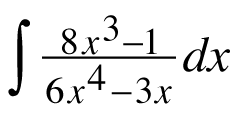2.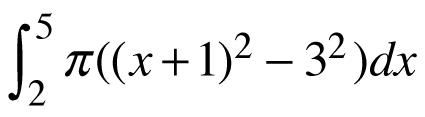3.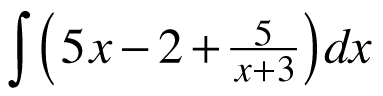4.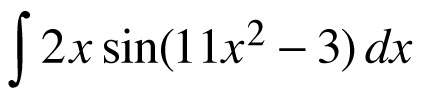5.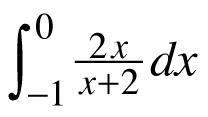6.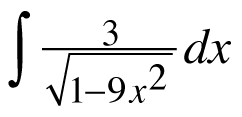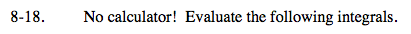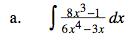U-substitution.

U = 6x4 − 3x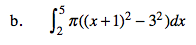Before you integrate, factor the π out of the integrand. You may (or may not) choose to expand (x + 1)².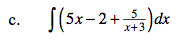$\text{Recall that }\frac{5}{x+3}\text{ is a transformation of }\frac{1}{x},$

$\text{ and you know the antiderivative of }\frac{1}{x}.$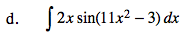U-substitution.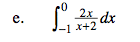You could long divide first or... use U-Substitution.
Let U = the denominator.

If U = x + 2 then x = U − 2.

$\text{Therefore numerator }2x=2(U-2) \text{ and }\frac{dU}{dx}=1 \text{ so }dU=dx.$

Also bounds U(0) = 2 and U(− 1) = 1
Rewrite the integral and evaluate.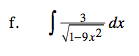Recall that 9x² = (3x
Then look for a familiar antiderivative.